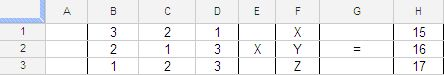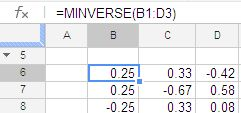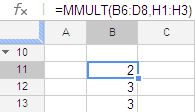## HOW TO SOLVE 3X3 MATRIX USING GOOGLE DOCS

Let say we want to solve the following equations for value of X, Y & Z

3X + 2Y + Z = 15

2X + Y + 3Z = 16

X + 2Y + 3Z = 17

if we convert this in matrix for the problem will look like thisWe using the two functions namely MINVERSE and MMULT

MINVERSE will calculate the inverse of the matrix and MMULT is used the derive the values of X, Y & Z valuethe formula in cell B6 is =MINVERSE(B1:D3)

which is nothing but the inverse of the matrix

now to solve the X, Y, Z we are using the MMULTthe formula in cell B11 is =MMULT(B6:D8,H1:H3)

so the solution to the problem is X=2, Y=3 & Z=3

you can also use the both in the same formula which will be =MMULT(MINVERSE(B1:D3),H1:H3)